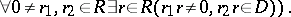# Fractions, ring of

(diff) ← Older revision | Latest revision (diff) | Newer revision → (diff)
A ring related to a given associative ringwith an identity. The (right classical) ring of fractions ofis the ringin which every regular element (that is, not a zero divisor) ofis invertible, and every element ofhas the form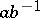with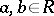. The ringexists if and only ifsatisfies the right-hand Ore condition (cf. Associative rings and algebras). The maximal (or complete) right ring of fractions ofis the ring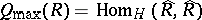, whereis the injective hull ofas a right-module, and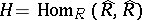is the endomorphism ring of the right-module. The ringcan also be defined as the direct limit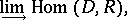whereis the set of all dense right ideals of(a right idealof a ringis called a dense ideal if